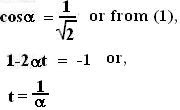## Tuesday, July 17, 2007

### Irodov Problem 1.25

(a) This part of the problem is exactly as the previous one. x=at gives us t = x/a which could then be substituted in the y equation to obtain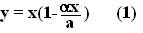(b)The point's position can be expressed as,The magnitudes of velocity and acceleration are then simply given by the Euclidean norms,(c)The angle between w and v is given by the vector dot product as below: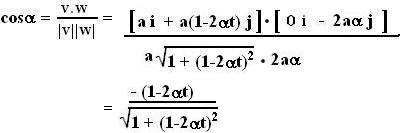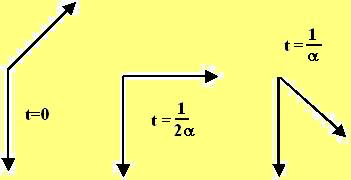Now, what does this mean physically? This is shown in the figure below. Basically, at time t=0, thev = a i + a j and is oriented at 45 degrees to the x-axis. The acceleration vector never changes its direction or magnitude with time and is oriented along the -ive y-axis. As time goes by, the ever persistent downward acceleration vector rotates the velocity vector v in the clockwise direction. At time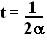, v aligns itself with the x-axis and is at 90 degrees to the w
vector. Finally the time when the angle between the two vectors is 45 degrees is given by,